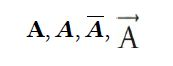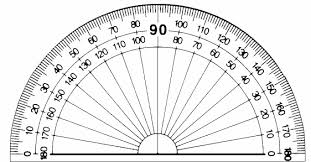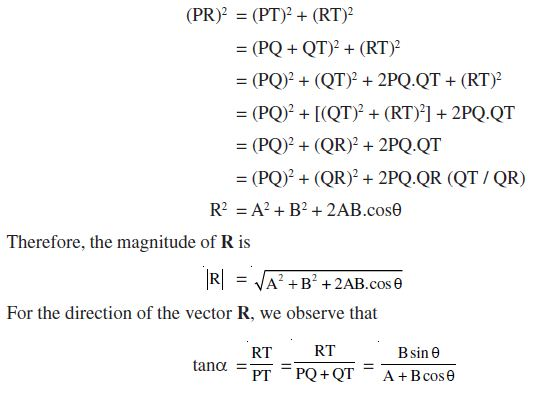# What is Vector Physics?

What is the vector physics chapter? And what we learn in this chapter?
Vector physics is one of the most fundamental chapters in high school physics where we learn about Vector and Scalar quantities.
To understand it we have to proceed step by step starting with physical quantity.

The quantity which can be measured is called physical quantity. The physical quantities measured in physics can be divided into two groups, scalars, and vectors.

## Scalar and Vector

Scalar Definition: Scalars are physical quantities that have a magnitude only. Examples of scalars are the length, speed, mass, density, energy, power, temperature, charge, and potential difference.

Vector Definition: Vectors in physics are physical quantities that have magnitude as well as direction. Examples of vector quantity are displacement, force, torque, velocity, acceleration, momentum, and electric current.

## What is Vector physics – definition, examples, and notation

What is vector physics? Define Vector?

Vectors in physics are physical quantities that not only have magnitude but also direction. Let’s take an example:

The quantity 55 km per hour is a scalar, while the quantity 55 km per hour to the east is a vector.

A vector can be graphically represented by a line with an arrowhead.
The length of the line represents the magnitude of the vector and the direction of the arrowhead indicates the direction of the vector.

Vectors can be denoted in several ways in text, including:In textbooks, you’ll generally see one of the first two, but when it’s handwritten, you’ll find one of the last two.

Scalars can be added together by simple arithmetic but when two or more vectors are added together then simple arithmetic is not sufficient, as their direction must be taken into account as well.

The addition of vectors gives us the resultant vector. This post is going to cover a wide area of vector physics. Apart from addition, we will gradually cover subtraction of vectors, vector resolution, vector product, vector dot product, vector cross product, etc. If you are looking for vector class 11 content for CBSE, ISC or state boards, you are in right place.

## Resultant Vector – Addition of vectors in physics

When two or more vectors are added the resulting sum of the vectors is called the resultant vector or simply the resultant. The resultant vector represents the net effect of the vectors added.

### Resultant of Vectors acting in the same line or parallel – what is vector physics

Two or more vectors acting in the same line (same direction or just opposite direction) can be added as if they were scalars.

Here Vectors may lie in the same line or may be parallel to each other.

For example, the sum, or resultant, of the two forces shown in figure (a) below is 500 N acting towards the right.

The resultant of the two forces shown in figure (b) below is 100 N towards right.

Again in figure (c) below, we added 3 vectors and the sum is 100N towards left.

### Resultant of Vectors acting in different directions – scale diagram and formula-based calculation

If the two vectors acting on a body are not acting along the same line then the resultant vector can’t be found so easily as the above-described cases.

This time the resultant can be found by either using a scale diagram(geometrical) or by calculation using vector addition formula.

Scale diagram:
A scaling factor is decided first (say 100 N of force =1 cm). Accordingly, 2 straight lines representing 2 vectors are drawn with specific ‘scaled’ length and direction.

The two vectors are drawn ‘head to tail’. This completes 2 sides of the triangle. The 3rd side is drawn now that closes the triangle.

This side represents the resultant vector. Its direction is taken from the Tail of the first vector to the head of the second vector. This is called the Law of Triangle for Vector Physics or Triangle law of vector addition.

### Triangle law of vector addition – what is vector physics

If two vectors are denoted by two sides of a triangle in sequence, then the third closing side of the triangle, in the opposite direction of the sequence, represents the sum or resultant of the two vectors in both magnitude and direction.

## How to solve vector problems in physics – find resultant of 2 vectors

In the above diagram (a) please find two vectors A (400 N) and B (300 N).
For simplicity, we have taken them at a right angle to each other.
Also pls note the directions of the 2 vectors from the arrowheads they have.

We have to find out the resultant of A and B. We will try both geometrical (scaling) way and the calculation using formula.

### Finding the Resultant vector using a Scale diagram – what is vector physics

#### setup

In diagram (a) above we see 2 vectors A and B.

For simplicity of calculation at this moment we have taken 2 vectors at a
right angle.

The magnitude of A and B vectors are 400 N and 300 N respectively.

We have to take a convenient scaling system, like for every 100 N in this case, we may take 1 cm.

#### Steps

Using this scaling (100 N = 1 cm) factor, we will draw a scale diagram in both magnitude and direction. We use a ruler and protractor.

See fig b now.

First, say we draw a line of 4 cm length using a ruler, representing 400 N and the direction is west to east as per the direction of vector A.

The arrowhead of vector A would be the point where tail of vector B will reside.
Accordingly, vector B is drawn as a line of length 3 cm (for 300 N) at a right angle with respect to the ‘vector A’ line and it stands at the head of the ‘vector A’ line.

Thus head to tail scaling representations are made for vector A and B.

We get the resultant vector R as we draw the line starting from the initial point(tail of vector A) and ending at the final point(head of vector B) that closes the triangle (Figure b).

If properly drawn, we will get the length of this line as 5cm which represents 500 N as per scaling factor used.

The direction of the resultant can be found by measuring angle θ between the resultant R and vector A. We use a protractor to measure the angle.In this case, θ = 37°.

So we get the resultant of vector A and B which is 500 N making an angle of 37° with vector A.

### Finding the resultant with formula of Pythagorean theorem – vector physics

The resultant R of the two vectors in figure (a) can also be found by calculation.
For this special case, when two vectors are at right angle, we can use the Pythagorean theorem to find out the R.

[In other cases when the angle between two vectors is different, we use a different formula. We will discuss this later in this tutorial.]
So, in this case, using Pythagorean theorem,
resultant R = √(4002 + 3002) = √250000 = 500 N

The direction of R can be found in the following manner:
tanθ  =300/400= 0.75
So, θ = 36.9°

Always we should choose a sensible scale when drawing scale diagrams of vectors.

vector

### Notebook

An example of two vectors not acting at right angles to each other is shown in Figure (c) and Figure(d). Please see below.

– A scaling diagram can be used to find out the resultant for the vector of fig c in the same fashion of fig a.

-However, to find out the resultant of vectors of fig c using calculations, we need to find out the formula to be used for these cases (as Pythagorean formula won’t work here), where vectors are not in right angle to each other. The Law of Parallelogram of vectors (discussed and derived below) comes to save us here.

### How to solve vector addition problems – when vectors are not at the right angle

#### Solution – Using Scale diagram hands-on approach @ Vector physics lab

Here we have adopted a hands-on approach as we take at our vector physics lab.

Say, a block is resting on a surface. Two forces A and B are working on the block as shown above.

The magnitude of force A is 400 N and the magnitude of force B is 350 N.

As per the diagram, the 400 N force is being applied parallel to the horizontal surface. And 350 N is being applied at an angle say 30 degree with the horizontal.

Now we have to find out the net force which is working on the block.
It is evident that the resultant force of the 2 forces is actually the net force that is being applied on the block.
Here you can see very easily that you just can’t use algebra to add the magnitudes of these 2 forces, as they are not parallel to each other.
So let’s use the Scale Diagram to find out the resultant or net force.

In the above diagram, we have separately drawn the two vectors according to a specific scaling factor. That factor is: 100 N is equivalent to 1 cm in our ruler.

So we draw a 4 cm horizontal line with an arrowhead towards east=> this is vector A (400N force)in our diagram.

And we also draw a 3.5 cm line making a 30 degree with the horizontal line => this represents vector B (350 N force) in our diagram.

##### vector class 11 – DIY approach @ Vector Physics Lab

Now you can see in the above diagram that 2 vector lines are brought together to form 2 sides of a triangle. But what is wrong with it?

Yes, you are right. These are added in a head-to-head fashion. But as per the Law of Triangle of vectors or triangle rule for vector physics, these are to be added head-to-tail fashion. So the vector B line is to be shifted without changing its direction and length as per the next diagram. (see below)

Head to tail – tail of B is touching the head of A in the above diagram.
Now its turn to close the triangle by adding its 3rd side.
This 3rd side is the resultant of vector A and B, as per the law of triangle of vectors or triangle law of vector physics. Here its direction is from the tail of vector A to head of vector B.

##### Measurement of the resultant vector (magnitude and direction)

Here R is the resultant as said earlier.

Now use your ruler to measure its length in cm. multiply this length with 100 (scaling factor taken) and get the magnitude of the resultant R.

Use a protractor to find the angle between R and A. It gives you the angle made by R with respect to a given vector A.

And the arrowhead shown in the above diagram also gives you complete info about its direction.

So now you have added 2 vectors and got the sum as the resultant vector. Students who are looking for content for vector class 11, hope this DIY stuff will give you a good grasp on this chapter. Note that this method is also termed as the head-to-tail graphical method of vector addition.

## Law of Polygon of vectors – Addition of three or more vectors in physics

If more than 2 vectors are to be added then we can use the extended version of ‘Law of triangle’. This extended version of the law is known as the Law of polygon of vectors.

According to this law, 3 or more vectors are connected using head to tail method (same as above: using vector lines of proportionate length and actual angle) as the sides of a polygon in a sequence.

Then the side which closes the polygon represents the resultant vector with its direction from the tail of the first vector to the head of the last vector added.

If we add the same vectors in a different order, the result will be the same. This is an important characteristic of vectors. The vector addition is commutative. Vectors can be added in any order.
A + B = B + A.

## Formula based approach – resultant vector physics

We have already seen that Scale diagram and law of triangle are nice tools to find out the resultant vector.

But probably all will agree that a ‘formula-based approach’ is a faster way of getting the same result.

In a section of this article, we have already used the Pythagorean theorem and its formula to find out the resultant when two vectors we added were at a right angle to each other.

But when the vectors we are adding are at a different angle then only Pythagorean theorem can’t help. Here comes the Law of Parallelogram of vectors. We will discuss this now.

### Law of Parallelogram of vectors

The parallelogram law of vectors states that if two adjacent sides of a parallelogram denote two vectors in magnitude and direction, then the diagonal of the parallelogram through the common point of the two sides represents the sum of the two vectors in both magnitude and direction.

#### Parallelogram Law of Vector Addition – What is Vector physics

Let A and B be the two vectors and let θ be the angle between them as shown in Fig above.
To calculate the vector sum, we complete the parallelogram.

Here side PQ represents vector A, side PS represents B and the diagonal PR represents the resultant vector R.
The diagonal PR is the sum vector A +B. It is called the resultant of vectors A and B.
The resultant makes an angle α with the direction of vector A.
Remember that vectors PQ and SR are equal to A, and vectors PS and QR are equal, to B.

To get the magnitude of the resultant vector R, drop a perpendicular RT as shown. Then in terms of magnitudes:So, the direction of the resultant can be expressed in terms of the angle it makes with base vector.

The above set of formulas to find out the magnitude and direction of the resultant vector is something a student should practice and remember if she/he wants to solve problems from the Vector chapter.

## Vector Subtraction – what is vector physics

Vector Subtraction helps us to subtract one vector from another vector and get the result in vector format. To find out the relative velocity of an object with respect to another moving object Vector subtraction procedure takes an important role.

Now, If we subtract vector B from vector A, then what can we do? Just see.

A – B = A + (-B)
This means the Addition of vector A with vector -B.
-B is nothing but the flipped version of vector B.
Thus we can use vector addition procedures in vector subtraction as well, but yes, only after flipping or reversing the direction of vector B.

In this post covering “What is Vector physics” we have made a deep dive to detail out the Vector concepts and Vector Addition laws and different mechanisms. We have also guided on ‘how to solve vector problems in physics’.

Vector Subtraction needs more detailed discussion and we have to study its applications as well.

Now as this post has become really long let’s move to the next post of this series and discuss Vector Subtraction. The link is below for that vital article. I love to see you there.

FYI:Vector is generally taught in length in class 11 for boards like ISC, CBSE and other state boards.

wiki

What is Vector Physics?
Scroll to top
error: protected !!